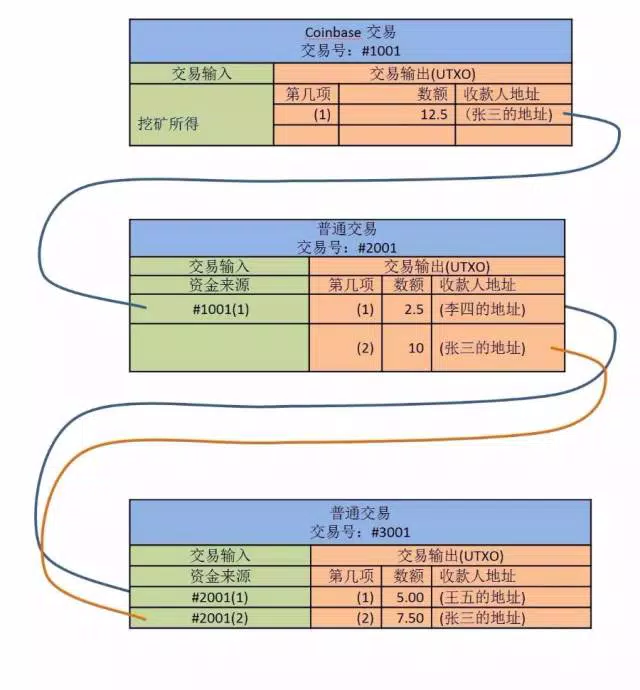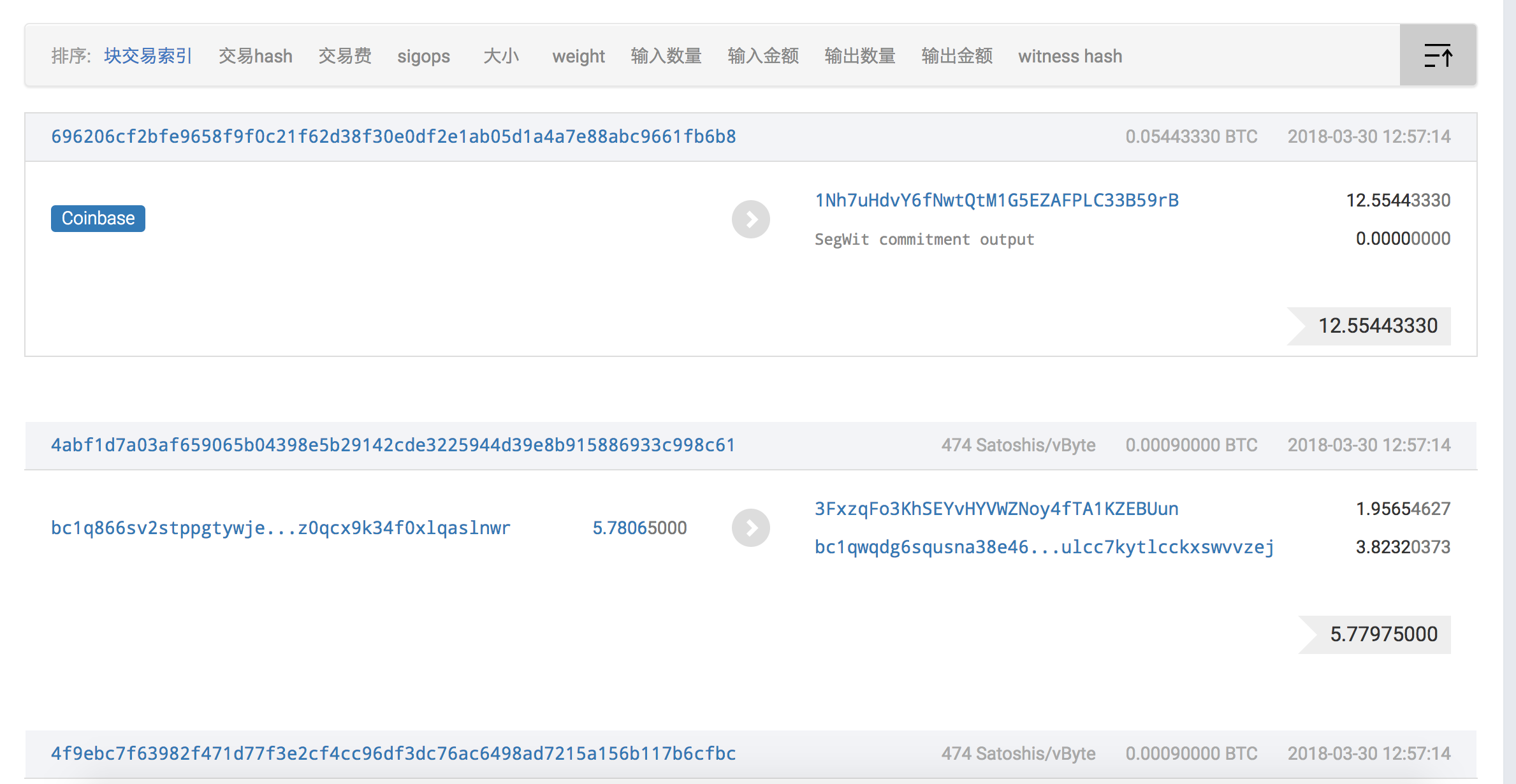# node.js与比特币（typescript实现）

## BTC中的utxo模型

BTC中引入了许多创新的概念与技术，区块链、PoW共识、RSA加密、萌芽阶段的智能合约等名词是经常被圈内人所提及，诚然这些创新的实现使得BTC变成了一种有可靠性和安全性保证的封闭生态系统，但是在这个BTC生态中如果没有搭配区块链模式的转账模块，那么货币的流通属性也就无从谈起了。若要实现转账交易模块， “是否采用传统的账户模型实现交易；如何在区块链上存储交易信息，如何实现信息压缩；如何验证交易信息；系统的最大交易并发量”等问题确实值得思考。

BTC一一解决了这些，它放弃了传统的基于账户的交易模型，而是采用基于区块链存储的utxo（unspent transaction output）模型。笔者尝试分析了为什么不使用传统的账户模型：

1. BTC的存储单元为区块链，区块链的数据结构本质上是单向链表，它并不是传统的关系型数据库，无法新建账户表
2. 存储压力。如果采用传统的方式，则账户表会随着时间的推移不停地增大，为后续的表的分片与备份造成很大困难
3. 易造成隐私泄露。账户表的信息会直观的暴露余额等敏感信息

utxo模型则很有技巧的避免了这些，在utxo模型下实现的每一笔交易，都不需要显式的提供转账地址和接收地址（utxo中没有账户，也不需要提供地址），只需提供这比交易的 交易输入交易输出 即可，而交易输入与交易输出又是什么？## UTXO的node.js实现

### 交易输入

export class Input {
private txId: string;
private outputIndex: number;
private unlockScript: string;

public get $txId(): string { return this.txId; } public set$txId(value: string) {
this.txId = value;
}

public get $outputIndex(): number { return this.outputIndex; } public set$outputIndex(value: number) {
this.outputIndex = value;
}

public get $unlockScript(): string { return this.unlockScript; } public set$unlockScript(value: string) {
this.unlockScript = value;
}

constructor(txId: string, index: number, unlockScript: string){
this.txId = txId;
this.outputIndex = index;
this.unlockScript = unlockScript;
}

// 反序列化，进行类型转换
public static createInputsFromUnserialize(objs: Array<Input>){
let ins = [];
objs.forEach((obj)=>{
ins.push(new Input(obj.txId,obj.outputIndex,obj.unlockScript));
});
return ins;
}

canUnlock (privateKey: string): boolean{
if(privateKey == this.unlockScript){
return true;
}else{
return false;
}
}
}


outputIndex表示 “这个可用的utxo在对应交易的序号值”；
unlockScript则是解锁脚本，此处并未完全按照BTC的原型去实现，而是简单的验证使用者的私钥来实现鉴权，原理上仍遵从BTC的思想。

### 交易输出

import * as rsaConfig from '../../rsa.json';
export class Output {
private value: number;
// 锁定脚本，需要使用UTXO归属者用私钥进行签名通过
// 当解锁UTXO成功后，此UTXO变为下一个交易的交易输入，同时使用接收方的地址（公钥）锁定本次交易的交易输出，
// 等待接收方使用私钥签名使用该UTXO
// 因此，btc没有账户的概念，所有的“钱”由自己的公钥所加密保存，只有用自己的私钥才能使用这些钱（即解锁了UTXO的解锁脚本）
private lockScript: string;

// 该属性仅仅在交易时使用，设置属性
private txId: string;

// 该属性仅仅在交易时使用，设置属性
private index: number;

public get $index(): number { return this.index; } public set$index(value: number) {
this.index = value;
}

public get $txId(): string { return this.txId; } public set$txId(value: string) {
this.txId = value;
}

public get $value(): number { return this.value; } public set$value(value: number) {
this.value = value;
}

/* public get $lockScript(): string { return this.lockScript; } public set$lockScript(value: string) {
this.lockScript = value;
} */

constructor(value: number,publicKey: string){
this.value = value;
this.lockScript = publicKey;
}

// 反序列化，进行类型转换
public static createOnputsFromUnserialize(objs: Array<Output>){
let outs = [];
objs.forEach((obj)=>{
outs.push(new Output(obj.value,obj.lockScript));
});
return outs;
}

public canUnlock(privateKey: string): boolean{
if(privateKey == rsaConfig[this.lockScript]){
return true;
}else{
return false;
}
}
}


lockScript属性为锁定脚本，在我们的简易实现中就为接收方的公钥，并不是BTC中的逆波兰式，但大体原理相同，都需要提供私钥来进行解密。

## 一笔交易

export class Transaction {
private txId: string;
private inputTxs: Array<Input>;
private outputTxs: Array<Output>;

constructor(txId: string, inputs: Array<Input>, outputs: Array<Output>){
this.txId = txId;
this.inputTxs = inputs;
this.outputTxs = outputs;
}

public get $txId(): string { return this.txId; } public set$txId(value: string) {
this.txId = value;
}

public get $inputTxs(): Array<Input> { return this.inputTxs; } public set$inputTxs(value: Array<Input>) {
this.inputTxs = value;
}

public get $outputTxs(): Array<Output> { return this.outputTxs; } public set$outputTxs(value: Array<Output>) {
this.outputTxs = value;
}
/*
1.交易结构各字段序列化为字节数组
2.把字节数组拼接为支付串
3.对支付串计算两次SHA256 得到交易hash
*/
public setTxId(){
let sha256 = crypto.createHash('sha256');
sha256.update(JSON.stringify(this.inputTxs) + JSON.stringify(this.outputTxs) + Date.now(),'utf8');
this.txId = sha256.digest('hex');
}

}


### coinbase交易

我们都知道得到比特币需要挖矿，其实挖矿也属于一种交易，不过是一种没有确定交易输入的一种交易，它也被称作coinbase交易。coinbase交易在每一个区块中都会存在，它的总额包括了系统针对矿工打包交易过程的奖励以及其他转账方提供的手续费，如下图：// coinbase交易用于给矿工奖励，input为空，output为矿工报酬
public static createCoinbaseTx(pubKey: string, info: string){
let input = new Input('',-1,info);
let output = new Output(AWARD, pubKey);
let tx = new Transaction('',[input],[output])
tx.setTxId();
return tx;
}


public static isCoinbaseTx(tx: Transaction){
if(tx.$inputTxs.length == 1 && tx.$inputTxs.$outputIndex == -1 && tx.$inputTxs.$txId == ''){ return true; }else{ return false; } }  至此，coinbase交易就完成了，这是最简单的一种交易，并没有涉及到转账方，也就是交易输入。 ### 转账交易 使用BTC就避免不了转账，转账事务在utxo模型的实现就是添加了一笔Transaction到某个区块而已。每一笔交易都需要交易输入和交易输出，因此在BTC中，转账的核心就是找到转账方的utxo进行消费，同时将指定数量的BTC划到指定的消费输出上，如果仍有剩余，则找零至自己的消费输出。 // 创建转账交易 public static createTransaction(from: string, fromPubkey: string, fromKey: string, to: string, toPubkey: string, coin: number){ let outputs = this.findUTXOToTransfer(fromKey, coin); console.log(UTXOToTransfer：${JSON.stringify(outputs)}, from: ${from} to${to} transfer ${coin}) let inputTx = [], sum = 0, outputTx = []; outputs.forEach((o)=>{ sum += o.$value;
inputTx.push(new Input(o.$txId,o.$index,fromKey));
});

if(sum < coin){
throw Error(余额不足，转账失败! from ${from} to${to} transfer ${coin}btc, but only have${sum}btc);
}

// 公钥锁住脚本
outputTx.push(new Output(coin,toPubkey));
if(sum > coin){
outputTx.push(new Output(sum-coin,fromPubkey));
}
let tx = new Transaction('',inputTx,outputTx);
tx.setTxId();
return tx;
}


接下来根据获得的可用utxo，进行创建对应的交易输入；

public static getAllUnspentOutputTx(secreteKey: string): Array<Transaction>{
let outputIndexHash: Object = this.getAllSpentOutput(secreteKey);
let unspentOutputsTx = [];
let keys = Object.keys(outputIndexHash);
let block = BlockDao.getSingletonInstance().getBlock(chain.$lastACKHash); while(block && block instanceof Block){ block.$txs && block.$txs.forEach((tx)=>{ if(keys.includes(tx.$txId)){
tx.$outputTxs.forEach((output,i)=>{ // 过滤已消费的output if(i == outputIndexHash[tx.$txId])
return;

if(output.canUnlock(secreteKey)){
unspentOutputsTx.push(tx);
}
});
}else{
for(let i=0,len=tx.$outputTxs.length;i<len;i++){ let output = tx.$outputTxs[i];
if(output.canUnlock(secreteKey)){
unspentOutputsTx.push(tx);
break;
}
}
}
});
block = BlockDao.getSingletonInstance().getBlock(block.\$prevHash);
}
return unspentOutputsTx;
}


## 尾声

posted @ 2018-03-30 13:50  royalrover  阅读(1623)  评论(3编辑  收藏  举报